7.1.4 The KIRKPATRICK Point Location MethodNext: Retriangulation Up: 7.1 GRS Previous: 7.1.3 Generic Interpolation

## 7.1.4 The KIRKPATRICK Point Location Method

The KIRKPATRICK point location method is also termed triangulation refinement method for point location [Kirk83][Prep85], because it is based on a hierarchy of triangulations. The initial grid makes up the lowest (finest) level of the hierarchy, and the upper levels are constructed as coarsened triangulations of the next lower level.

A precondition for applying the KIRKPATRICK method is that the lowest level has to be a triangulation. This is not always the case, but there exist algorithms to triangulate any given point set consisting ofpoints in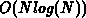time [Prep85][Hala94]. According to the EULER formula, such a triangulation of a vertex set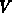has at mostedges.

Surrounding the initial grid, a bounding triangle is assumed where the initial triangulation is inscribed. This has the effect that the top level triangulation consists of only this one triangle.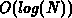locate time is only guaranteed with this precondition.

Let the grid hierarchy be a sequence of triangulations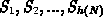, whereis the initial-vertex triangulation. The method to obtain the triangulation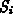from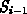is shown in Algorithm 7.1.This sequence of triangulations is now stored as a search-directed acyclic graph (SDAG), which is a tree structure whose nodes are triangles. Fig. 7.9 shows how a triangulation is coarsened through vertex removal, and Fig. 7.10 shows the corresponding SDAG.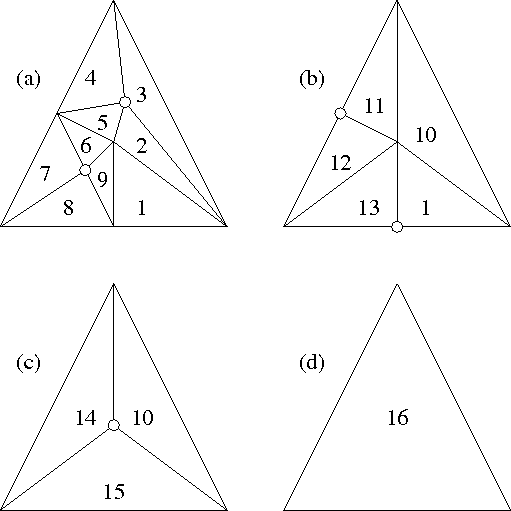Figure: Coarsening the initial triangulation through point removal. Case (a) shows an initial triangulation consisting of 9 rectangles which is coarsened in four steps. Points with a degree of less than five (constantis chosen to be five) are marked for removal and shown encircled. Note that in case (b) boundary points lying on a straight line are selected.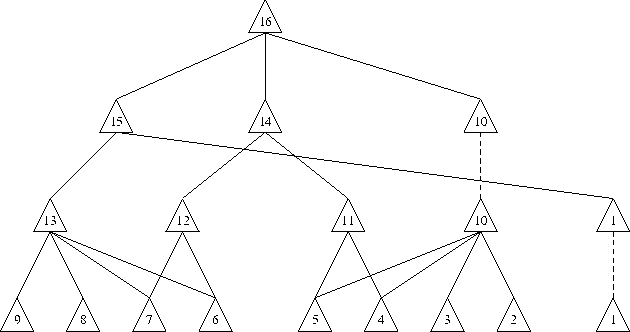Figure: The resulting search-directed acyclic graph. Solid lines denote direct references. Dashed lines denote promotion of nodes. Sincewas chosen to be five, at most four nodes are referenced by inner nodes.

In this implementation of the KIRKPATRICK point location method, three deviations from Algorithm 7.1 are made:

• The initial grid need not necessarily be a triangulation; the point removal step is applied to any unstructured element.

• For now, no surrounding triangle is constructed for the initial grid; this does affect performance, especially on complicated geometries with many boundary points. However, boundary points lying on a straight line are selectable for removal, because they do not contribute to the overall shape of the geometry. Those points would not occur at all if there were a surrounding triangle.

• Triangles (elements) which are not touched during a point removal step are promoted to the next newly constructed hierarchy. This simplifies the internal memory structures and has the additional advantage of an always consistent grid hierarchy which can even be written to PIF for further processing.

Multiply connected regions in two dimensions are handled correctly, because if no surrounding triangle is generated, the top-level grid always matches exactly the geometry. Only in the case of a surrounding triangle, inner nodes would be generated which refer to ``void'' triangles, i.e. triangles not included in the initial geometry. If a point location would encounter such a void triangle, the point is not included in the initial grid and the location process is done.

Point location in the SDAG now proceeds according to Algorithm 7.2. Initially, the questioned point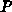is tested for inclusion in the triangulation. The test for inclusion in a triangle to be used throughout the location process can be carried out in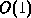time. If the point is included in the root triangle, then it is tested for inclusion in one of its descendants. This process is repeated until a triangle belonging tois reached.Next: Retriangulation Up: 7.1 GRS Previous: 7.1.3 Generic Interpolation

Martin Stiftinger
Tue Nov 29 19:41:50 MET 1994